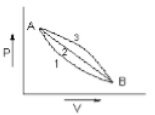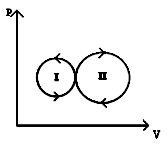You’ve reached the end of your free Videos limit.
#21 | Work (W) done by the Gas: 2
(Physics) > Thermodynamics
Unable to watch the video, please try another server
Related Practice Questions :

A given mass of gas expands from state A to state B by three paths 1,2 and 3 as shown in the figure. If respectively be the work done by the gas along the three paths then1.

2.

3.

4.

High Yielding Test Series + Question Bank - NEET 2020

Difficulty Level:

For the indicator diagram given below to select the wrong statement.1.  Cycle - II is a heat engine cycle

2.   Net work is done on the gas in cycle - I

3.   Work done is positive for the cycle I

4.  Work done is positive for the cycle - II

High Yielding Test Series + Question Bank - NEET 2020

Difficulty Level:

If ${W}_{1}$ is the work done in compressing an ideal gas from a given initial state through a certain volume isothermally and ${W}_{2}$ is the work done in compressing the same gas from the same initial state through the same volume adiabatically, then:

(1) ${W}_{1}={W}_{2}$

(2) ${W}_{1}<{W}_{2}$

(3) ${W}_{1}>{W}_{2}$

(4) ${W}_{1}=2{W}_{2}$

High Yielding Test Series + Question Bank - NEET 2020

Difficulty Level:

A gas is compressed isothermally to half its initial volume. The same gas is compressed separately through an adiabatic process until its volume is again reduced to half .Then -

(a) compressing the gas through adiabatic process will require more work to be done
(b) compressing the gas isothermally or adiabatically will require the  same amount work
(c) which of the case (whether compression through isothermal or through adiabatic process) requires more work will depend upon the atomicity of the gas
(d) compressing the gas isothermally will require more work to be done

High Yielding Test Series + Question Bank - NEET 2020

Difficulty Level:

An ideal gas is compressed to half its initial volume by means of several process. Which of the process results in the maximum work done on the gas?